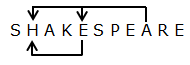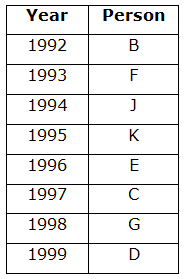# RRB Clerk Mains Reasoning Ability Questions 2021 (Day-04)

Dear Aspirants, Our IBPS Guide team is providing new series of Reasoning Questions for RRB Clerk Mains 2021 so the aspirants can practice it on a daily basis. These questions are framed by our skilled experts after understanding your needs thoroughly. Aspirants can practice these new series questions daily to familiarize with the exact exam pattern and make your preparation effective.

#### Click Here to – Get SBI PO Online Practice Mock Test

Start Quiz

Number series- conditions based

Direction (1-4): Study the following information carefully and answer the below questions:

There are two rows given. To find out the resultant of a particular row we need to follow the below conditions.

Condition 1: If an odd number is followed by an even number, then the second number is subtracted from the first number.

Condition 2: If an odd number is followed by another odd number then both the numbers are to be added.

Condition 3: If an even number is followed by another even number, then the second number is the resultant number.

Condition 4: If an even number is followed by an odd number, then both the numbers are to be multiplied.

Condition 5: If the final resultant number of a row is even, then it should be divided by 2.

Condition 6: If the final resultant number of a row is odd, then subtract 1 from the resultant number.

1) If x is the resultant of the second row, then find the resultant of the first row?

Row 1:  45        16 x

Row 2: 32 55 14

a) 42

b) 38

c) 36

d) 18

e) None of these

2) Find the resultant of the second row where Y is the resultant of row 1.

Row 1: 49 18    33

Row 2: 26 Y     57

a) 872

b) 458

c) 912

d) 946

e) None of these

3) Find the resultant of the two final results of both rows.

Row 1: 56 17    34

Row 2: 45 71    23

a) -1312

b) -1318

c) -1348

d) 2668

e) -1456

4) If the sum of the resultant of the two rows is 18, then what is the value of z (Note: exclude conditions 5 and 6)?

15     21     z

31     42     17

a) 24

b) 12

c) 18

d) 36

e) None of these

Misc

5) How many such pairs of letters are there in the word “SHAKESPEARE” which have as many letters between them as there are in the English alphabetical series (both forward and backward)?

a) One

b) Two

c) Three

d) More than three

e) None

Number series

Direction (6-9): Study the following information carefully and answer the below questions:

3248 2397 5724 7415 3276

6) If the number is odd, then reverse the two digits in the middle and if it is even, add 2 to the first digit of the number, then which of the number thus formed is the second lowest?

a) 2397

b) 3248

c) 5724

d) 7415

e) 3276

7) If all the digits are arranged in descending order within the number from left to right, then which of the following is the difference between the highest and the lowest number?

a) 2119

b) 2190

c) 2151

d) 2191

e) None of these

8) If the second digit of the number is divisible by 2, then interchange the first and third digits else interchange the second and fourth digit within the number and arranging all the numbers in ascending from left to right, then which of the following will be the second from the right end?

a) 5724

b) 3276

c) 7415

d) 3248

e)2397

9) How many numbers is/are there in the number series between the third digit of the second number from the left end and second digit of the third number from the right end?

a) Four

b) Two

c) One

d) Three

e) None

Year puzzle

10) Eight persons viz. B, C, D, E, F, G, J and K are born on different years from 1992 to 1999, but not necessarily in the same order. E was born in a leap year and two years before G who was born immediately after C. Four persons were born between C and B who was born immediately before F. J was born in an even-numbered year immediately before K. D was not born before J. Who among the following person was the youngest?

a) D

b) F

c) K

d) G

e) None of these

45     16     x

32     55     14

In row 2, as per condition 4, 32 and 55 are multiplied. 32 * 55 = 1760.

In row 2, as per condition 3, 14 is the resultant of row 2.

As per condition 5, the resultant is divided by 2. Hence, x = 14/2 = 7.

45     16     7

In row 1, as per condition 1, 16 is subtracted from 45. 45 – 16 = 29.

In row 1, as per condition 2, 29 is added to 7. 29 + 7 = 36.

As per condition 5, the resultant is divided by 2. Hence, 36/2 = 18 is the answer.

49     18     33

26     Y      57

In row 1,

As per condition 1, 18 is subtracted from 49. 49 – 18 = 31.

As per condition 2, 31 is added to 33. 31 + 33 = 64.

As per condition 5, 64 is divided by 2. 64 / 2 = 32. Hence Y = 32.

26     32     57

In row 2,

As per condition 3, 32 is considered as the resultant.

As per condition 4, 32 is multiplied with 57. 32 * 57 = 1824.

As per condition 5, 1824 is divided by 2. 1824 / 2 = 912.

56     17     34

45     71     23

In row 1,

As per condition 4, 56 is multiplied with 17. 56 * 17 = 952.

As per condition 3, 34 is considered as the resultant.

As per condition 5, 34 is divided by 2. 34 / 2 = 17.

45     71     23

In row 2,

As per condition 2, 45 is added to 71. 45 + 71 = 116.

As per condition 4, 116 is multiplied with 23. 116 * 23 = 2668.

As per condition 5, 2668 is divided by 2. 2668 / 2 = 1334.

From row 1 and 2, 17  1334

As per condition 1, subtract 1334 from 17. 17 – 1334 = -1317.

As per condition 6, 1 is subtracted from -1317. -1317 – 1 = -1318.

15     21     z

31     42     17

In row 2,

As per condition 1, 42 is subtracted from 31. 31 – 42 = -11.

As per condition 2, -11 is added to 17. -11 + 17 = 6.

Subtracting 6 from 18, 18 – 6 = 12 should be the resultant of row 1.

In row 1,

As per condition 2, 15 is added to 21. 15 + 21 = 36.

36     z => 12

On comparing the two values, 12 (resultant of row 1) and 36 (resultant of 15 and 21), by substitution method and considering the conditions, the value of z can be 12, as per condition 3.Hence, option C is correct.

3248 2397 5724 7415 3276

5248 2937 7724 7145 5276

Hence, option B is correct.

3248 2397 5724 7415 3276

8432 9732 7542 7541 7632

Hence, option D is correct.

3248 2397 5724 7415 3276

4238 2793 5427 1475 7236

1475 2793 4238 5427 7236

Hence, option A is correct.

3248 2397 5724 7415 3276

Hence, option C is correct.